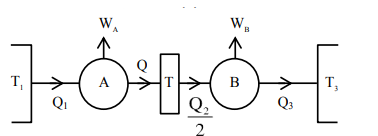# Two Carnot engines A and B operate in series such that engine A absorbs heat

Question:

Two Carnot engines A and B operate in series such that engine $A$ absorbs heat at $T_{1}$ and rejects heat to a sink at temperature $T$. Engine $B$ absorbs half of the heat rejected by Engine A and rejects heat to the sink at $\mathrm{T}_{3}$. When workdone in both the cases is equal, to value of $T$ is :

1. $\frac{2}{3} \mathrm{~T}_{1}+\frac{3}{2} \mathrm{~T}_{3}$

2. $\frac{1}{3} \mathrm{~T}_{1}+\frac{2}{3} \mathrm{~T}_{3}$

3. $\frac{3}{2} \mathrm{~T}_{1}+\frac{1}{3} \mathrm{~T}_{3}$

4. $\frac{2}{3} \mathrm{~T}_{1}+\frac{1}{3} \mathrm{~T}_{3}$

Correct Option: , 4

Solution:$\mathrm{W}_{\mathrm{A}}=1-\frac{\mathrm{Q}_{2}}{\mathrm{Q}_{1}}=1-\frac{\mathrm{T}}{\mathrm{T}_{1}} \Rightarrow \frac{\mathrm{Q}_{2}}{\mathrm{Q}_{1}}=\frac{\mathrm{T}}{\mathrm{T}_{1}}$

$\mathrm{W}_{\mathrm{B}}=1-\frac{\mathrm{Q}_{3}}{\left(\mathrm{Q}_{2} / 2\right)}=1-\frac{\mathrm{T}_{3}}{\mathrm{~T}} \Rightarrow \frac{2 \mathrm{Q}_{3}}{\mathrm{Q}_{2}}=\frac{\mathrm{T}_{3}}{\mathrm{~T}}$

Now, $\mathrm{W}_{\mathrm{A}}=\mathrm{W}_{\mathrm{B}}$

$\mathrm{Q}_{1}-\mathrm{Q}_{2}=\frac{\mathrm{Q}_{2}}{2}-\mathrm{Q}_{3}$

$\Rightarrow \frac{2 Q_{1}}{Q_{2}}+\frac{2 Q_{3}}{Q_{2}}=3$

$\Rightarrow \frac{2 T_{1}}{T}+\frac{T_{3}}{T}=3$

$\frac{2 T_{1}}{3}+\frac{T_{3}}{3}=T$